# 一文带你入门图论和网络分析

+关注继续查看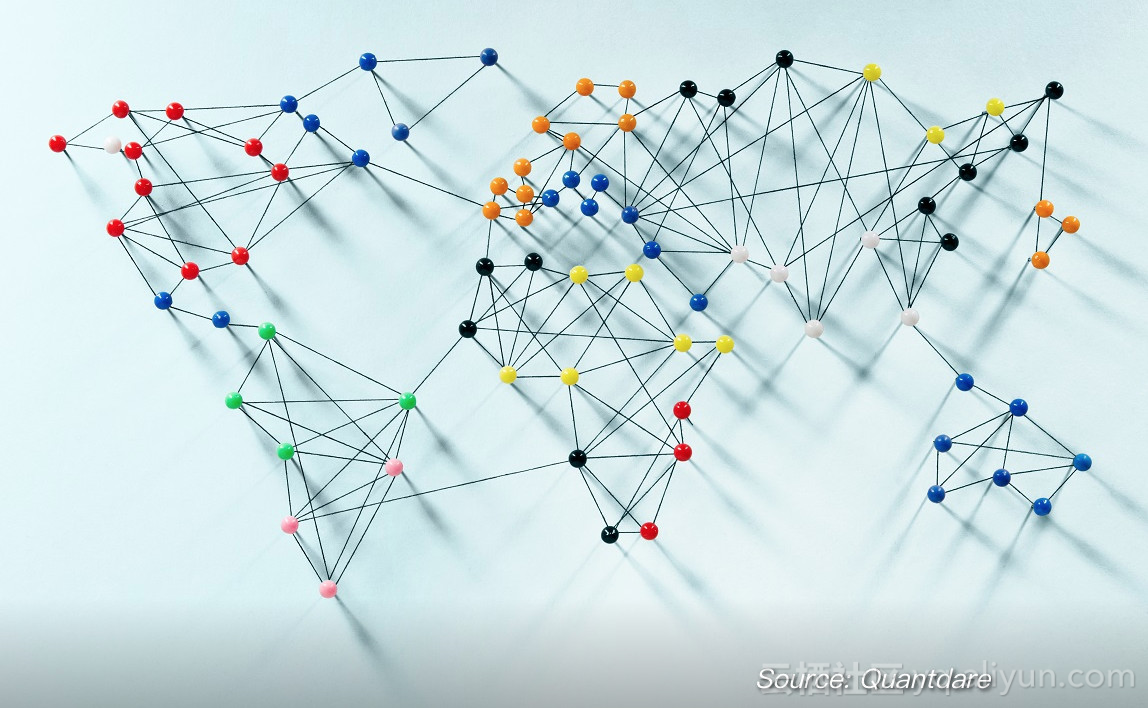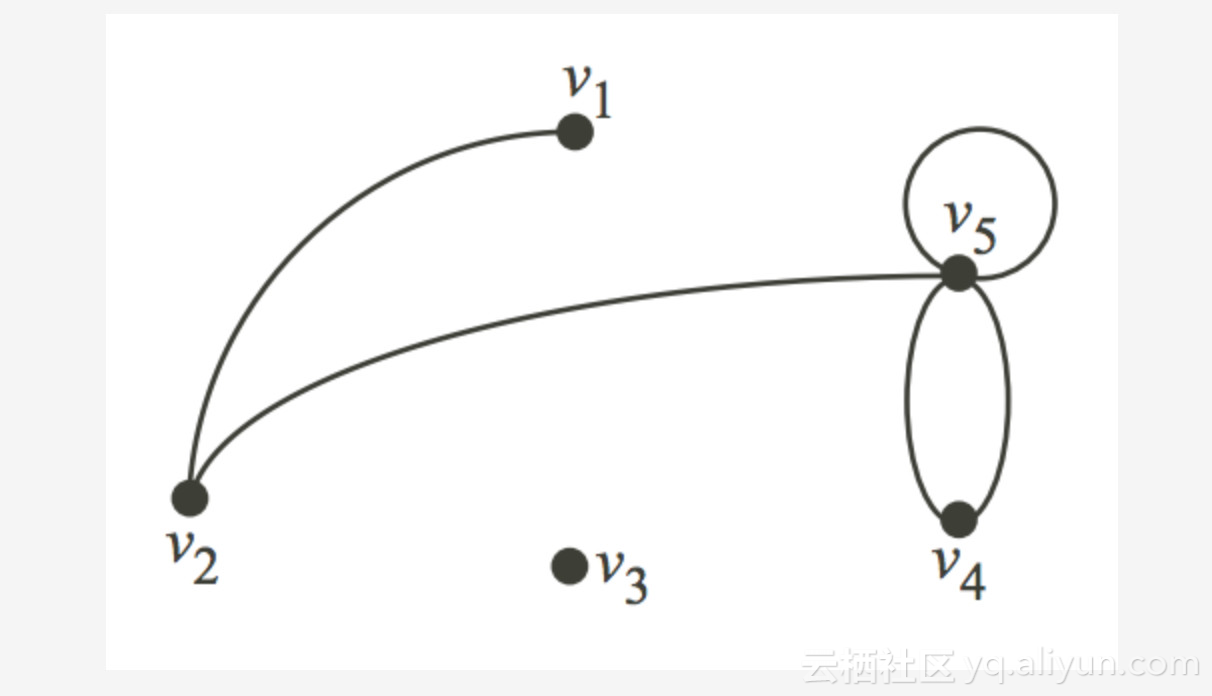V = {v1, v2, v3, v4, v5}

E = {(v1,v2), (v2,v5), (v5, v5), (v4,v5), (v4,v4)}


R和Python中都有使用图论概念分析数据的包。在本文中，我们将简要介绍一些概念并使用Networkx Python包分析一个数据集。

from IPython.display import Image
Image('images/network.PNG')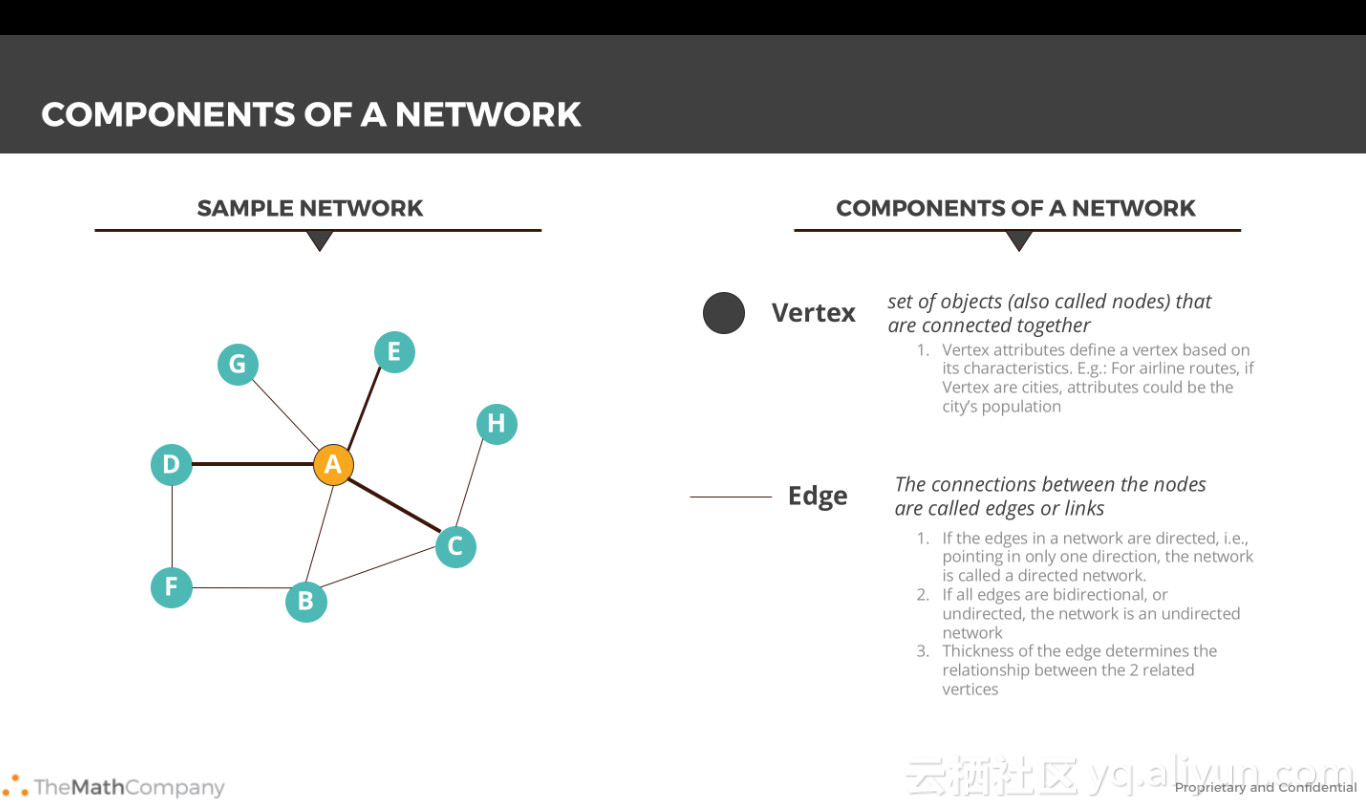Image('images/usecase.PNG')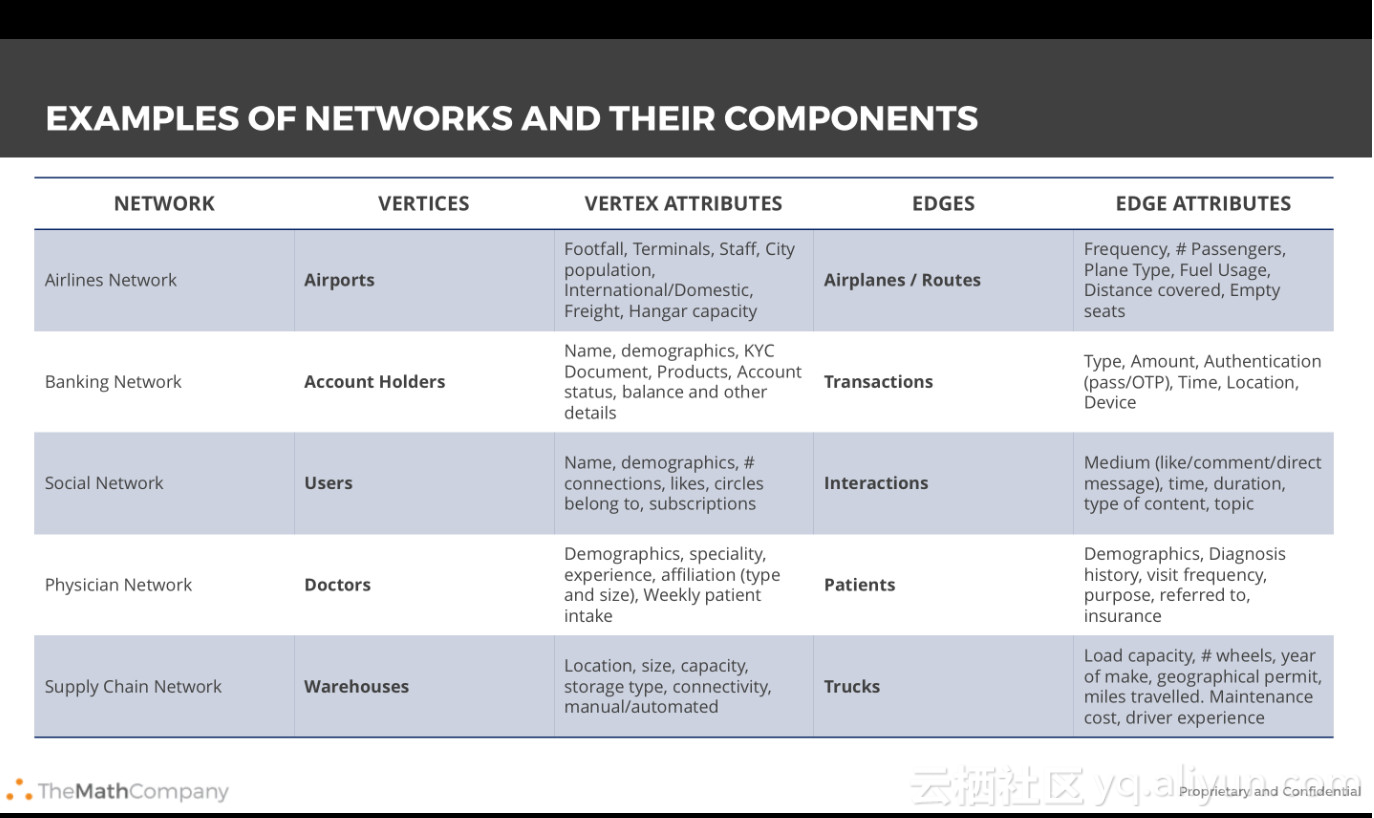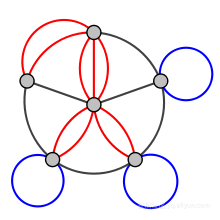1840年，A.F Mobius提出了完全图（complete graph）和二分图（bipartite graph）的概念，Kuratowski通过趣味谜题证明它们是平面的。树的概念（没有环的连通图）由Gustav Kirchhoff于1845年提出，他在计算电网或电路中的电流时使用了图论思想。

1852年，Thomas Gutherie发现了著名的四色问题。然后在1856年，Thomas P. Kirkman和William R.Hamilton研究了多面体的循环，并通过研究仅访问某些地点一次的旅行，发明了称为哈密顿图的概念。1913年，H.Dudeney提到了一个难题。尽管发明了四色问题，但Kenneth Appel和Wolfgang Haken在一个世纪后才解决了这个问题。这一次被认为是图论真正的诞生。

Caley研究了微分学的特定分析形式来研究树。这在理论化学中有许多含义。这也导致了枚举图论（enumerative graph theory）的发明。不管怎么说，“图”这个术语是由Sylvester在1878年引入的，他在“量子不变量”与代数和分子图的协变量之间进行了类比。

1941年，Ramsey致力于着色问题，这产生了另一个图论的分支 - 极值图论（Extremal graph theory）。1969年，Heinrich使用计算机解决了四色问题。对渐近图连通性的研究产生了随机图论。图论和拓扑学的历史也密切相关，它们有许多共同的概念和定理。

Image('images/Konigsberg.PNG', width = 800)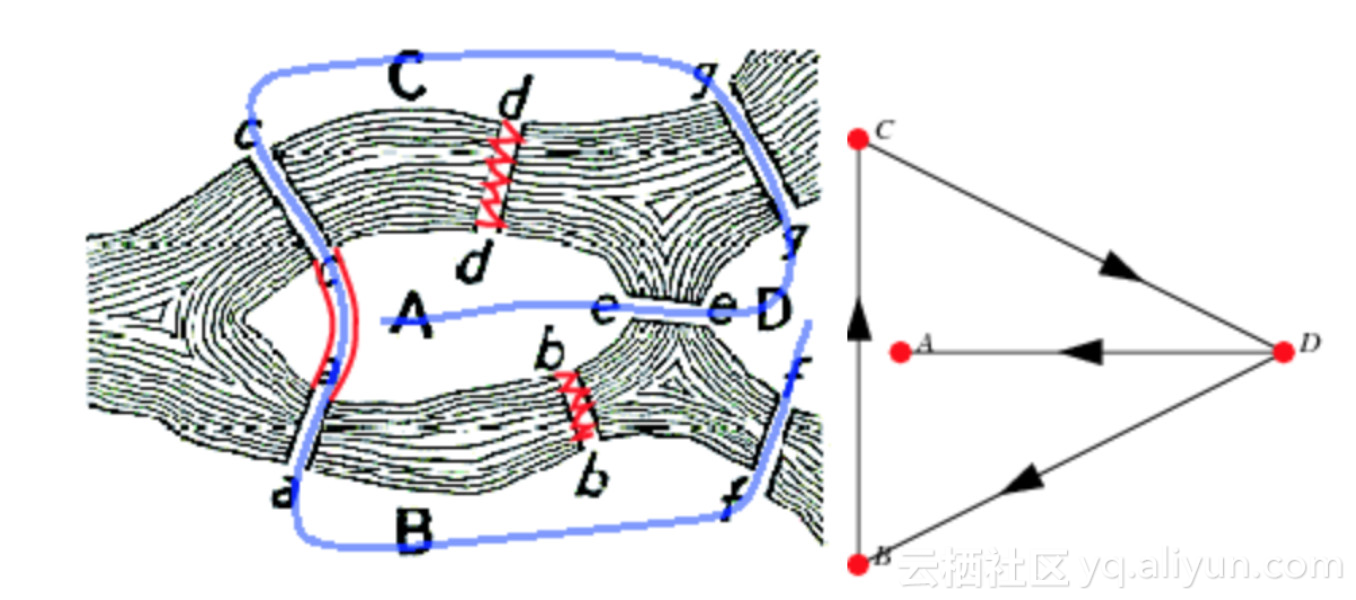BFS和DFS

BFS的目的是尽可能接近根节点遍历图，而DFS算法旨在尽可能远离根节点。

import networkx as nx

# Creating a Graph
G = nx.Graph() # Right now G is empty

G.add_nodes_from([2,3]) # You can also add a list of nodes by passing a list argument

e = (2,3)
G.add_edge(*e) # * unpacks the tuple
G.add_edges_from([(1,2), (1,3)]) # Just like nodes we can add edges from a list


subgraph(G, nbunch)      - induced subgraph view of G on nodes in nbunch
union(G1,G2)             - graph union
disjoint_union(G1,G2)    - graph union assuming all nodes are different
cartesian_product(G1,G2) - return Cartesian product graph
compose(G1,G2)           - combine graphs identifying nodes common to both
complement(G)            - graph complement
create_empty_copy(G)     - return an empty copy of the same graph class
convert_to_undirected(G) - return an undirected representation of G
convert_to_directed(G)   - return a directed representation of G


Image('images/graphclasses.PNG', width = 400)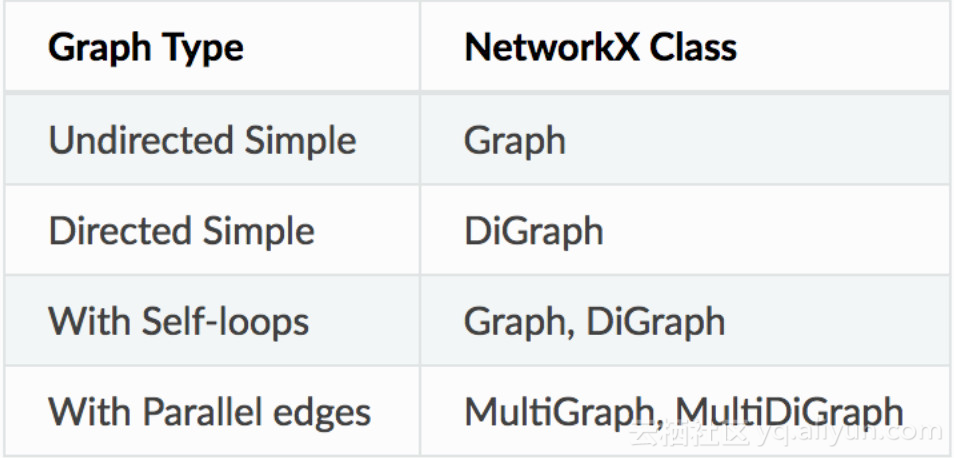G.nodes()
NodeView((1, 2, 3))
G.edges()
EdgeView([(1, 2), (1, 3), (2, 3)])
AtlasView({2: {}, 3: {}})
G
{}
G.edges[1, 2]
{}


Networkx提供了可视化图的基本功能，但其主要目标是帮助图分析而不是图的可视化。图可视化很难，我们将使用专门用于此任务的工具。Matplotlib提供了一些便利功能。但是GraphViz可能是最好的工具，因为它提供了一个PyGraphViz的Python接口（链接在文档的末尾）。

%matplotlib inline
import matplotlib.pyplot as plt
nx.draw(G)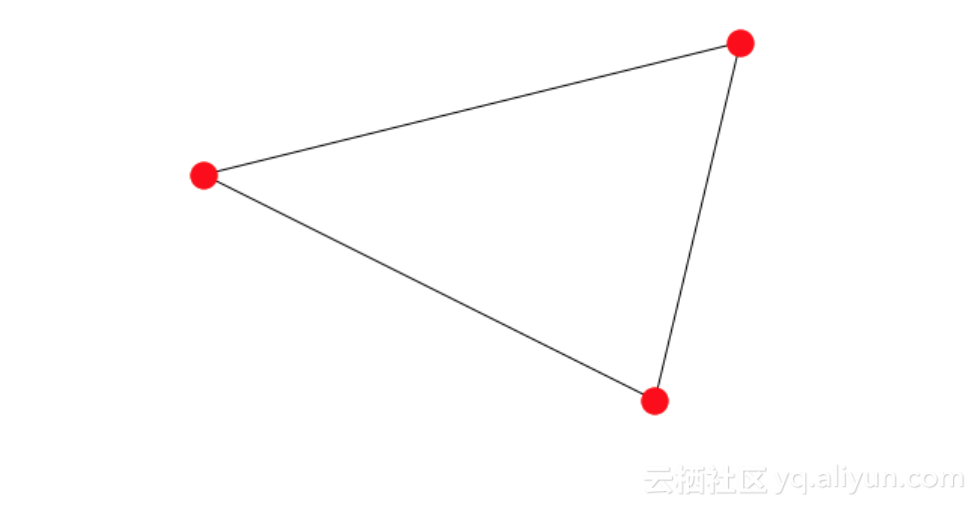import pygraphviz as pgv
d={'1': {'2': None}, '2': {'1': None, '3': None}, '3': {'1': None}}
A = pgv.AGraph(data=d)
print(A) # This is the 'string' or simple representation of the Graph

Output:

strict graph "" {
1 -- 2;
2 -- 3;
3 -- 1;
}


PyGraphviz可以很好地控制边和节点的各个属性。我们可以使用它获得非常漂亮的可视化。

# Let us create another Graph where we can individually control the colour of each node
B = pgv.AGraph()
# Setting node attributes that are common for all nodes
B.node_attr['style']='filled'
B.node_attr['shape']='circle'
B.node_attr['fixedsize']='true'
B.node_attr['fontcolor']='#FFFFFF'

# Creating and setting node attributes that vary for each node (using a for loop)
for i in range(16):
n=B.get_node(i)
n.attr['fillcolor']="#%2x0000"%(i*16)
n.attr['height']="%s"%(i/16.0+0.5)
n.attr['width']="%s"%(i/16.0+0.5)
B.draw('star.png',prog="circo") # This creates a .png file in the local directory. Displayed below.

Image('images/star.png', width=650) # The Graph visualization we created above.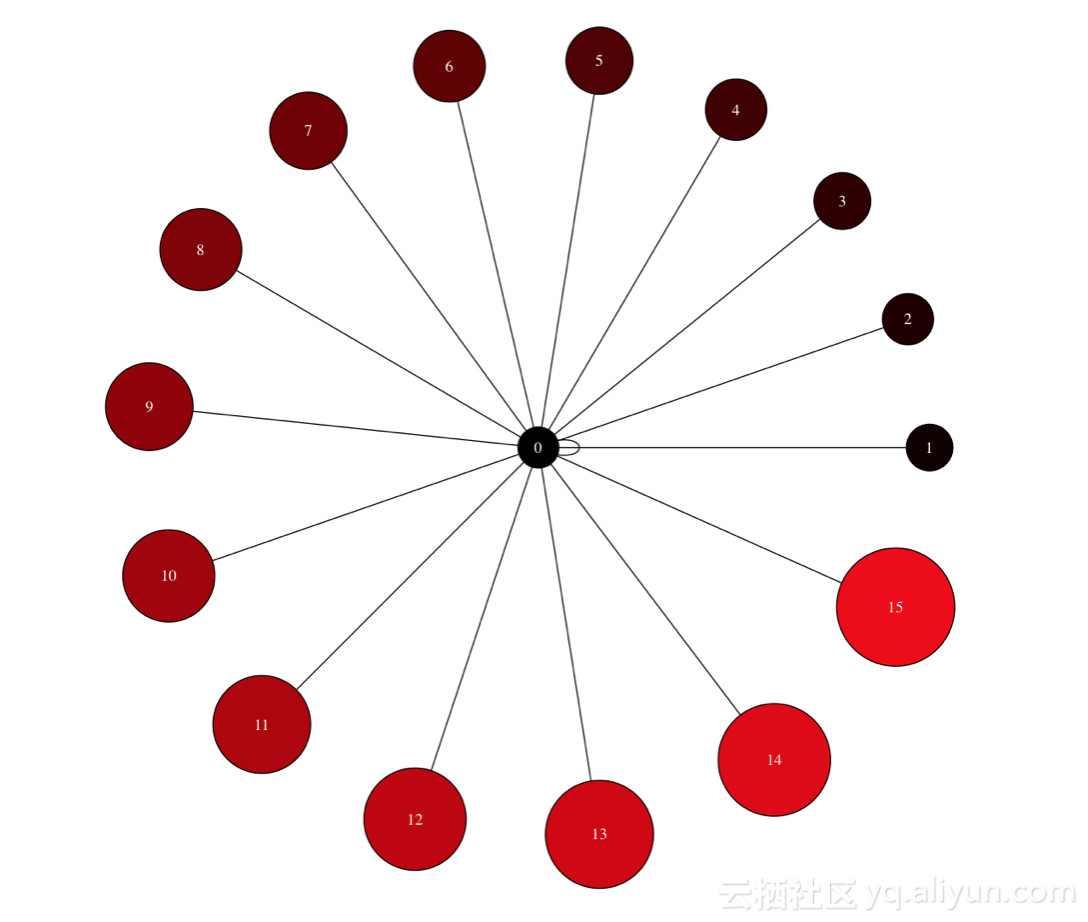import pandas as pd
import numpy as np

data.shape
(100, 16)

data.dtypes

year                int64
month               int64
day                 int64
dep_time          float64
sched_dep_time      int64
dep_delay         float64
arr_time          float64
sched_arr_time      int64
arr_delay         float64
carrier            object
flight              int64
tailnum            object
origin             object
dest               object
air_time          float64
distance            int64
dtype: object


# converting sched_dep_time to 'std' - Scheduled time of departure
data['std'] = data.sched_dep_time.astype(str).str.replace('(\d{2}$)', '') + ':' + data.sched_dep_time.astype(str).str.extract('(\d{2}$)', expand=False) + ':00'

# converting sched_arr_time to 'sta' - Scheduled time of arrival
data['sta'] = data.sched_arr_time.astype(str).str.replace('(\d{2}$)', '') + ':' + data.sched_arr_time.astype(str).str.extract('(\d{2}$)', expand=False) + ':00'

# converting dep_time to 'atd' - Actual time of departure
data['atd'] = data.dep_time.fillna(0).astype(np.int64).astype(str).str.replace('(\d{2}$)', '') + ':' + data.dep_time.fillna(0).astype(np.int64).astype(str).str.extract('(\d{2}$)', expand=False) + ':00'

# converting arr_time to 'ata' - Actual time of arrival
data['ata'] = data.arr_time.fillna(0).astype(np.int64).astype(str).str.replace('(\d{2}$)', '') + ':' + data.arr_time.fillna(0).astype(np.int64).astype(str).str.extract('(\d{2}$)', expand=False) + ':00'


data['date'] = pd.to_datetime(data[['year', 'month', 'day']])

# finally we drop the columns we don't need
data = data.drop(columns = ['year', 'month', 'day'])


import networkx as nx
FG = nx.from_pandas_edgelist(data, source='origin', target='dest', edge_attr=True,)

FG.nodes()


NodeView(('EWR', 'MEM', 'LGA', 'FLL', 'SEA', 'JFK', 'DEN', 'ORD', 'MIA', 'PBI', 'MCO', 'CMH', 'MSP', 'IAD', 'CLT', 'TPA', 'DCA', 'SJU', 'ATL', 'BHM', 'SRQ', 'MSY', 'DTW', 'LAX', 'JAX', 'RDU', 'MDW', 'DFW', 'IAH', 'SFO', 'STL', 'CVG', 'IND', 'RSW', 'BOS', 'CLE'))

FG.edges()


EdgeView([('EWR', 'MEM'), ('EWR', 'SEA'), ('EWR', 'MIA'), ('EWR', 'ORD'), ('EWR', 'MSP'), ('EWR', 'TPA'), ('EWR', 'MSY'), ('EWR', 'DFW'), ('EWR', 'IAH'), ('EWR', 'SFO'), ('EWR', 'CVG'), ('EWR', 'IND'), ('EWR', 'RDU'), ('EWR', 'IAD'), ('EWR', 'RSW'), ('EWR', 'BOS'), ('EWR', 'PBI'), ('EWR', 'LAX'), ('EWR', 'MCO'), ('EWR', 'SJU'), ('LGA', 'FLL'), ('LGA', 'ORD'), ('LGA', 'PBI'), ('LGA', 'CMH'), ('LGA', 'IAD'), ('LGA', 'CLT'), ('LGA', 'MIA'), ('LGA', 'DCA'), ('LGA', 'BHM'), ('LGA', 'RDU'), ('LGA', 'ATL'), ('LGA', 'TPA'), ('LGA', 'MDW'), ('LGA', 'DEN'), ('LGA', 'MSP'), ('LGA', 'DTW'), ('LGA', 'STL'), ('LGA', 'MCO'), ('LGA', 'CVG'), ('LGA', 'IAH'), ('FLL', 'JFK'), ('SEA', 'JFK'), ('JFK', 'DEN'), ('JFK', 'MCO'), ('JFK', 'TPA'), ('JFK', 'SJU'), ('JFK', 'ATL'), ('JFK', 'SRQ'), ('JFK', 'DCA'), ('JFK', 'DTW'), ('JFK', 'LAX'), ('JFK', 'JAX'), ('JFK', 'CLT'), ('JFK', 'PBI'), ('JFK', 'CLE'), ('JFK', 'IAD'), ('JFK', 'BOS')])

nx.draw_networkx(FG, with_labels=True) # Quick view of the Graph. As expected we see 3 very busy airports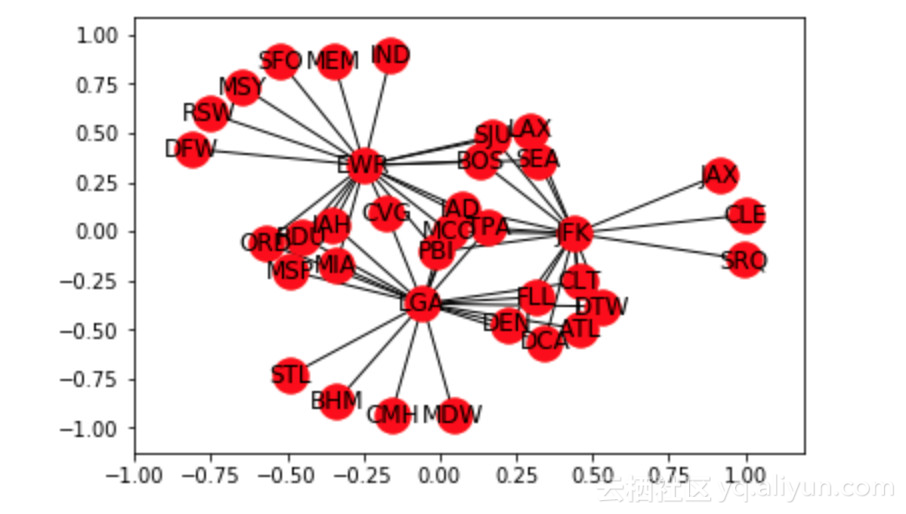nx.algorithms.degree_centrality(FG) # Notice the 3 airports from which all of our 100 rows of data originates
nx.density(FG) # Average edge density of the Graphs


0.09047619047619047

nx.average_shortest_path_length(FG) # Average shortest path length for ALL paths in the Graph


2.36984126984127

nx.average_degree_connectivity(FG) # For a node of degree k - What is the average of its neighbours' degree?


{1: 19.307692307692307, 2: 19.0625, 3: 19.0, 17: 2.0588235294117645, 20: 1.95}


# Let us find all the paths available
for path in nx.all_simple_paths(FG, source='JAX', target='DFW'):
print(path)
# Let us find the dijkstra path from JAX to DFW.
# You can read more in-depth on how dijkstra works from this resource - https://courses.csail.mit.edu/6.006/fall11/lectures/lecture16.pdf
dijpath = nx.dijkstra_path(FG, source='JAX', target='DFW')
dijpath


['JAX', 'JFK', 'SEA', 'EWR', 'DFW']

# Let us try to find the dijkstra path weighted by airtime (approximate case)
shortpath = nx.dijkstra_path(FG, source='JAX', target='DFW', weight='air_time')
shortpath


['JAX', 'JFK', 'BOS', 'EWR', 'DFW']|
12天前
|

14 0
|
12天前
|

8 0
|
11月前
|

R 语言是为数学研究工作者设计的一种数学编程语言，主要用于统计分析、绘图、数据挖掘。 机器学习是一门多领域交叉学科，涉及概率论、统计学、逼近论、凸分析、算法复杂度理论等多门学科。专门研究计算机怎样模拟或实现人类的学习行为，以获取新的知识或技能，重新组织已有的知识结构使之不断改善自身的性能。
271 0|
11月前
|

R 语言是为数学研究工作者设计的一种数学编程语言，主要用于统计分析、绘图、数据挖掘。 机器学习是一门多领域交叉学科，涉及概率论、统计学、逼近论、凸分析、算法复杂度理论等多门学科。专门研究计算机怎样模拟或实现人类的学习行为，以获取新的知识或技能，重新组织已有的知识结构使之不断改善自身的性能。
151 1|
11月前
|

R 语言是为数学研究工作者设计的一种数学编程语言，主要用于统计分析、绘图、数据挖掘。 机器学习是一门多领域交叉学科，涉及概率论、统计学、逼近论、凸分析、算法复杂度理论等多门学科。专门研究计算机怎样模拟或实现人类的学习行为，以获取新的知识或技能，重新组织已有的知识结构使之不断改善自身的性能。
237 0